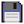Keys (API)

The Keys API provides a table of numerical codes corresponding to keyboard keys, suitable for decoding key events.Keys (API)
Function Return values Description
keys.getName(number code) string keyName Translates a numerical key code to a human-readable name.

Although this API contains only one method, it also contains a vast number of constants, one per key, containing the numerical key codes for those keys. As of ComputerCraft 1.52, the following constants are defined:

• a
• b
• c
• d
• e
• f
• g
• h
• i
• j
• k
• l
• m
• n
• o
• p
• q
• r
• s
• t
• u
• v
• w
• x
• y
• z
• one
• two
• three
• four
• five
• six
• seven
• eight
• nine
• zero
• minus
• equals
• backspace
• tab
• leftBracket
• rightBracket
• enter
• leftCtrl
• semiColon
• apostrophe
• grave
• leftShift
• backslash
• comma
• period
• slash
• rightShift
• multiply
• leftAlt
• space
• capsLock
• f1
• f2
• f3
• f4
• f5
• f6
• f7
• f8
• f9
• f10
• numLock
• scollLock
• f11
• f12
• f13
• f14
• f15
• kana
• convert
• noconvert
• yen
• cimcumflex
• at
• colon
• underscore
• kanji
• stop
• ax
• rightCtrl
• rightAlt
• pause
• home
• up
• pageUp
• left
• right
• end
• down
• pageDown
• insert
• delete

Note that when using the "end" key, you will have to write it as keys['end'] instead of keys.end, as otherwise it will be detected as the Lua keyword end and cause a script error.